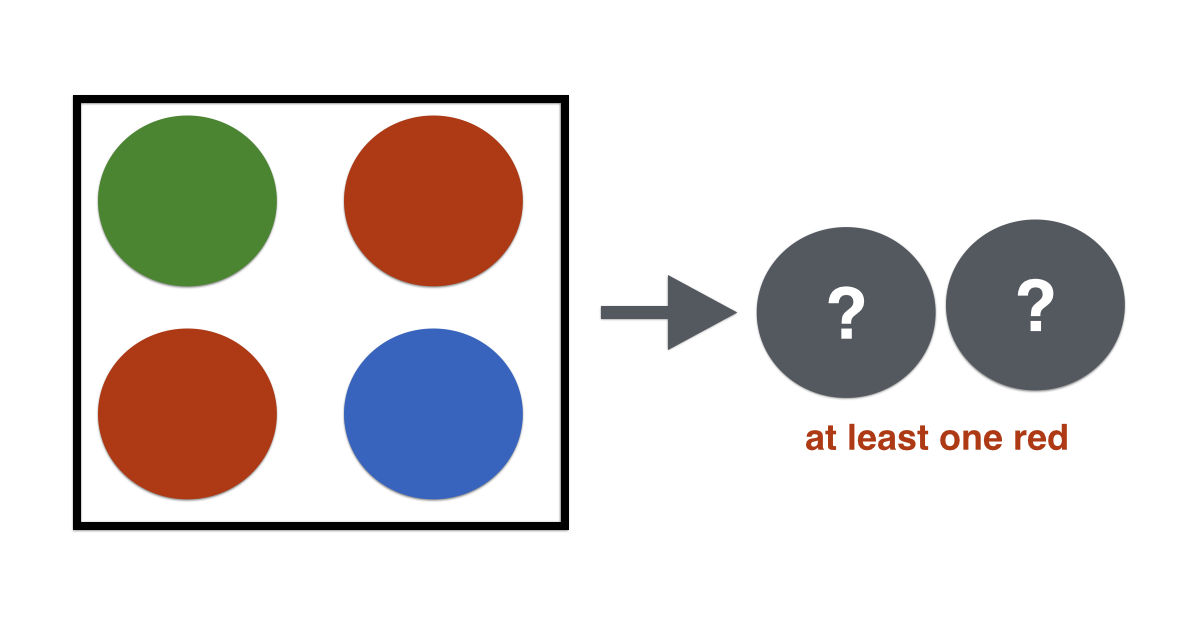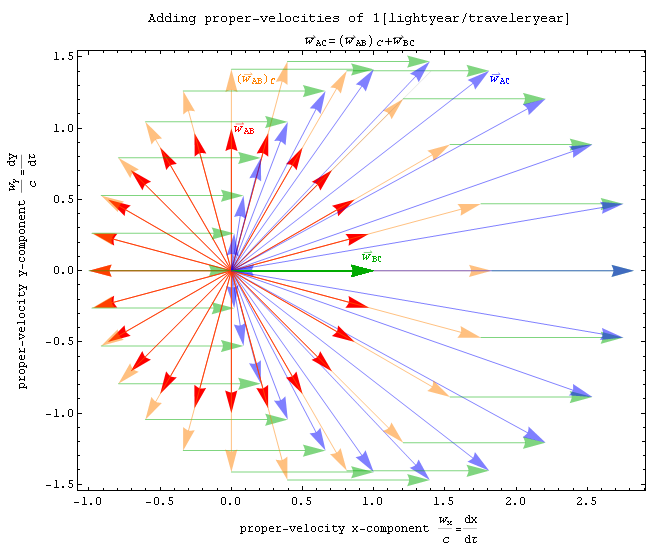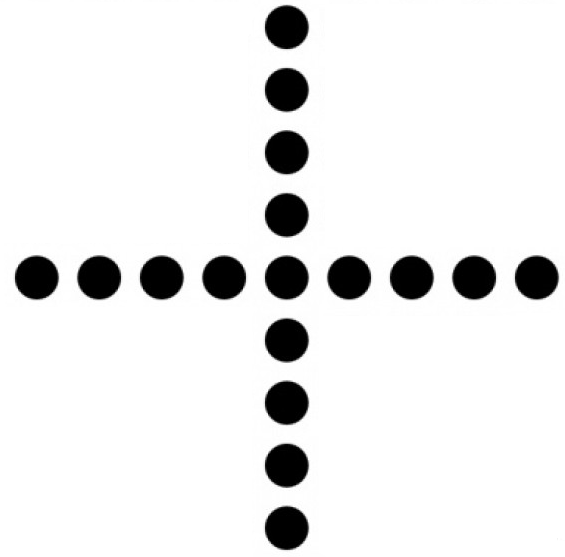Quantitative Finance

# Probability Theory: Level 4 Challenges

All the 7-digit numbers containing each of the digits 1, 2, 3, 4, 5, 6, 7 exactly once, and not divisible by 5, are arranged in the increasing order. Find the 2000th number in this list.One green ball, one blue ball, and two red balls are placed in a bowl. I draw two balls simultaneously from the bowl and announce that at least one of them is red.

What is the chance that the other ball I have drawn out is also red?Two unit vectors in two-dimensional space $\hat{\textbf{a}}$ and $\hat{\textbf{b}}$ are added together. The expected magnitude of the resulting vector $\textbf{a+b}$ is equal to $E.$ What is $\lfloor100E\rfloor?$

Also try Daniel Liu's Expected Distance on a Circle.

###### Image credit: Wikipedia P.Fraundorf

Five couples like to go to the movies together; they always sit in a row of ten adjacent seats. To shake things up a bit, they have a rule that nobody is allowed to sit next to their partner. How many seating arrangements are there for this party?

I thought of this problem while watching a bad (Hollywood) movie on a bad date ;)How many non-degenerate triangles can be formed with these dots?

×